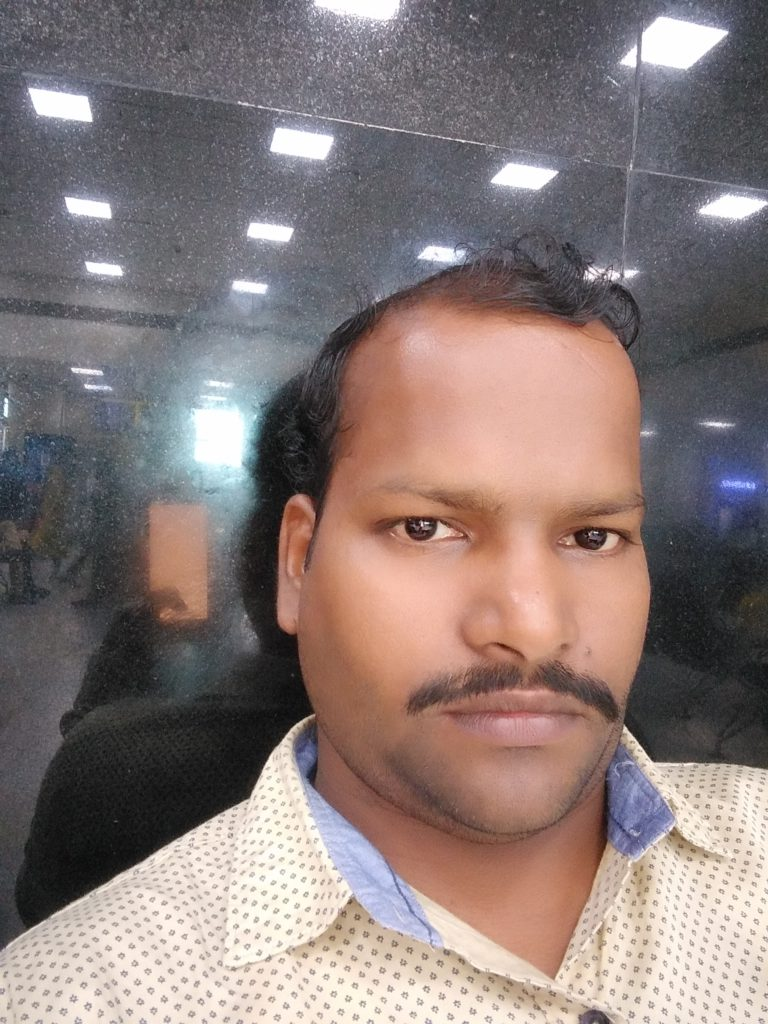# Partial Differential Equations And System of Ordinary Differential EquationsTeacherCategory

### Course Attendees

Still no participant

Still no reviews

# Code(Credit) : CUTM1517(3-2-1)

## Course Objectives

• Introduce students to partial differential equations.
• Introduce students to how to solve linear and non-linear Partial Differential Equations with different methods.
• To practice heat and wave equations in 2D and 3D..

## Learning Outcomes

Upon successful completion of this course, students will be able to:
• solve linear partial differential equations of both first and second order
• apply partial derivative equation techniques to predict the behavior of certain phenomena.
• apply specific methodologies, techniques and resources to conduct research and produce innovative results in the area of specialization.
• extract information from partial derivative models in order to interpret reality.

## Course Syllabus

Module-I(4 h + 4 h + 2 h)

Linear Homogeneous and Non-homogeneous system of Differential Equations with constant coefficients.

Practice-1

To find the general solution of linear homogeneous system of differential equations by using Matlab.

Practice-2

To find the general solution of linear non-homogeneous system of differential equations by using Matlab.

Project-1
To make a report of an application of linear system of differential equation

Module-II(4 h+ 4 h + 2 h )

Simultaneous linear first order differential equations in three variables, Pfaffian differential equations, Methods of Solutions of Pfaffian differential equations in three variables.

Practice-3
To find the solution of simultaneous linear first order differential equations by using Matlab.

Practice-4
To find the Solutions of system of differential equations in three Variables by using Matlab.

Project-2
Tomake a report on simultaneous Linear Differential Equations.

Module-III(4 h + 4 h)

Introduction to first order partial differential equations, formation of first order partial differential equations, linear Partial differential equations.

Practice-5
To find the solution of first order partial differential equations by using Matlab.

Practice-6
To find the solution of first order Quasi-linear partial differential equations by using Matlab.

Module-IV(5 h + 4 h +2 h)

Non-linear partial differential equations, Special types of first-order equations, Charpit Equation, solutions of partial differential equations of first order satisfying given initial conditions.
Practice-7

To find the solution of 1-d Wave equation by using Matlab.
Practice-8

To find the solution of 1-d Heat equation by using Matlab.

Project-3

Summary of Special types of partial Differential Equations.

Module-V(4 h + 2 h + 2 h)

First order homogeneous PDE with constant coefficients, higher order homogeneous PDE with constant coefficients.

Practice-9
To find the solution of first order homogeneous partial differential equations by using Matlab.

Project-4

Briefly describe Homogeneous Partial differential Equations with suitable examples.

Module-VI( 6 h + 2 h+ 2 h+)

Higher order linear Non-homogeneous PDE with constant coefficients, general Solution of PDE by using inverse operators.

Practice-10
To find the solution of 2-d Wave equation by using Matlab.

Project-5

Make a report on Non-homogeneous Partial differential Equations with constant coefficients.

Module-VII( 3 h + 4 h+ 2 h)

Solution of homogeneous and non-homogeneous PDE with variable coefficients.

Practice-11
To find the solution of 2-d heat equation by using Matlab.

Practice-12
To find the solution of 2-d Laplace equation by using Matlab.

Project-6

summary of Non-homogeneous Partial differential Equations with variable coefficients.

Text Book:

1. J. Sinha Roy and S. Padhy, A course on ordinary and partial differential equation, Kalyani Publisers, New Delhi, Ludhiana, 2012.

Reference Books:
1. Loknath Sahoo, Calculus and ordinary differential equations, Kalyani Publication, Reprint-2017
2. TynMyint-U and Lokenath Debnath, Linear Partial differential Equations for Scientists and Engineers, 4th Edition, Springer, Indian reprint, 2006
3. S.L. Ross, Differential Equations, 3rd Ed., John Wiley and Sons, India, 2004

4.Paul DuChateau, David W. Zachmann - Schaum’s Outline of Theory and Problems of Partial Differential Equations-McGraw-Hill.

## Session 4 & 5

Practice-1

To find the general solution of linear homogeneous system of differential equations by using Matlab

>

## Session 6

Non-Homogeneous Linear Systems with Constant Coefficients
https://player.slideplayer.com/26/8588018/#

## Session 7 & 8

Practice-2

To find the general solution of linear non-homogeneous system of differential equations by using Matlab
https://in.mathworks.com/videos/solving-odes-in-matlab-8-systems-of-equations-117652.html

## Session 9 & 10

Project-1

To make a report of an application of linear system of differential equation

## Session 11

Introduction to Simultaneous Linear First Order Equations in Three Variables
https://www.slideshare.net/shubhijain1042/simultaneous-differential-equations

## Session 13 & 14

Practice-3

To find the solution of simultaneous linear first order differential equations by using Matlab.

## Session 16

Methods of Solutions of Pfaffian Differential Equations in Three Variables.
pfaffian PDE

## Session 17 & 18

Practice-4

To find the Solutions of system of differential equations in three Variables by using Matlab.

## Session 19 & 20

Project-2

To make a report on simultaneous Linear Differential Equations.

## Session 24 & 25

Practice-5

To find the solution of first order partial differential equations by using Matlab

## Session 27 & 28

Practice-6

To find the solution of first order Quasi-linear partial differential equations by using Matlab

https://in.mathworks.com/help/matlab/math/partial-differential-equations.html

## Session 29 & 30

Project-3

Summary of Special types of partial Differential Equations.

Assignment-2

## Session 33 & 34

Practice-7

To find the solution of 1-d Wave equation by using Matlab

https://in.mathworks.com/help/pde/ug/wave-equation.html

## Session 40 & 41

Project-4

Briefly describe Homogeneous Partial differential Equations with suitable examples.

Assignment-3

## Session 43 & 44

Practice-9

To find the solution of first order homogeneous partial differential equations by using Matlab

## Session 46 & 47

Practice-10

To find the solution of 2-d Wave equation by using Matlab

https://www.slideshare.net/AmrMousa12/2-dimensional-wave-equation-analytical-and-numerical-solution

## Session 50 & 51

Project-5

Make a report for the Non-homogeneous Partial differential Equations.

## Session 55 & 56

Practice-11

To find the solution of 2-d heat equation by using Matlab

## Session 59 & 60

Practice-12

To find the solution of 2-d Laplace equation by using Matlab

## Session 61 & 62

Project-6:

summary of Non-homogeneous Partial differential Equations with variable coefficients.

## Case Studies